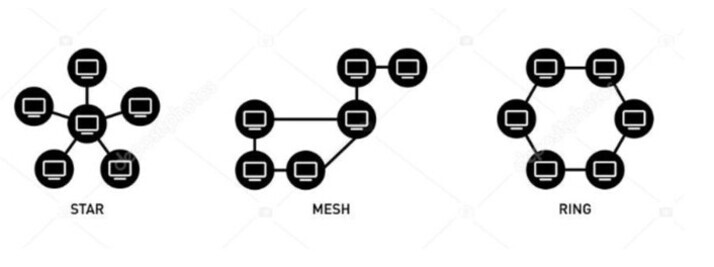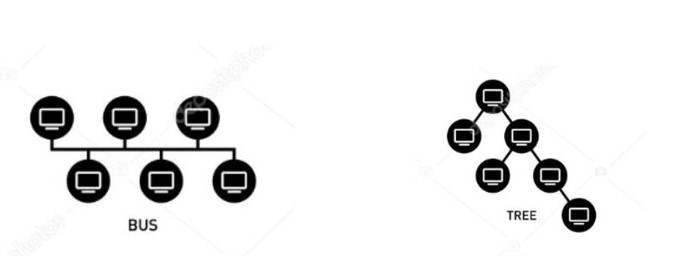# Types of Network Topology

The topology means that the elements are arranged in a particular order. They are divided into two type’s namely physical and logical topology. The nodes in the network are connected physically and the information flows through the network logically. In a computer network, the node is a point that connects data from one device to another and is used to transfer and receive information. Some of the physical nodes in the network are printers, routers, and wireless base stations and they provide an overall map of the network. There are also some logical nodes like the servers that include information about the physical node. Various types of network topology are covered in this article.

## Network Topology

Network Topology visually represents the network design. The main importance of using the network topology is to define the arrangements of the elements in different communication networks. The overall performance of the networks is calculated and also calculates the efficiency of the topology.

## Types of Network Topology

The Network topology is classified based on either the physical connections or the logical connections. The Physical connections include the layout of the communication network namely the Ethernet or the fibre optic cable. The Logical connections include the pattern in which the data is transferred. Primarily the network topology is divided into two types,

• Physical Network Topology

• Logical Network Topology

## Physical Network Topology

Physical topology generally means the interconnection of the LANs (Local Area Network). There are six types of physical network topology.

### Bus Topology

In this topology, all the nodes would be attached to a single cable or called Bus in a linear pattern. So, the transmission of data between the systems occurs only through this single bus. The Transmission of data is from one end to another end. The pictorial representation of the bus topology is shown below −

### Pros of Bus Topology

• The number of cables − The number of cables needed for connection is less.

• Cost − So, it provides the minimum budget for implementation.

• Function − Bus Topology is the simplest one compared to other topologies.

### Cons of Bus Topology

• The number of connections − Number of connections made is limited and data flow linearly.

• Data flow − As it uses only a single bus, it cannot transmit and receive data simultaneously which is unidirectional data transmission.

• Security − They also have less secure connection, as one node can hear the data that are sent to other nodes as they are connected to a single bus.

### Star Topology

The star network has a central node or hub that connects all other child nodes to it. The data is transmitted from the central hub to all other nodes. The Central hub controls all the nodes in the network. The pictorial representation after all the connections of nodes in LANs will be like a star.

### Pros of Star Topology

• Malfunction of a node − If the network fails or any one of the nodes is failed, that node is ignored and all other nodes will function properly.

• Performance − Overall performance is high due to the small network and traffic congestion.

### Cons of Star Topology

• Bottleneck − The main flaw is that they are prone to bottleneck and failure problems means that the central system slowdowns and traffic congestion occurs.

• Cost − The setup cost of Star topology is high.

### Ring Topology

In this topology, nodes are connected in a ring pattern or a closed-loop fashion. Every node is connected to two other nodes through a coaxial cable. The data flow from one node to another or transmits and receives data simultaneously.So the Ring network is bidirectional. There are two types of Ring topology, namely

• Counter-rotated ring topology

• Collapsed ring topology

Example − Optical Fibre

### Pros of Ring Topology

• Server − There is no need for a central hub to control other nodes.Packet Collision: Data flows from one node to another node, which is unidirectional that avoids packet collision.

### Cons of Ring Topology

• Performance − Data must pass through every node, so the performance is reduced.

• Cost − More Expensive

### Mesh Topology

It is a type of network in which all nodes are interconnected. The data flow from a short distance to reach the other node. There are many routes in this topology but does not have a hub or a single bus. Internet is a mesh topology.

There are two types of mesh topology namely,

• Full mesh topology

• Partial mesh topology

### Pros of Mesh Topology

• Multiple connections − As multiple connections are implemented, the data flow rate is high

• Troubleshooting − Troubleshooting is easier in this topology.

### Cons of Mesh Topology

• Cost − The cost of installation and maintenance is expensive

• Cable − The number of cables needed is more.

### Tree Topology

It has a root node and connects to the branches of the node, so it flows the Top-down approach. It is also known as hierarchical topology or star-Bus Topology as it combines the functions of both stars and bus topology.

### Pros of Tree Topology

• Connections − Any number of child nodes can be added to the parent node.

• Structure − The Entire structure is easier to construct.

### Cons of Tree Topology

• Cost − It is expensive as it must maintain more cables and child nodes.

• Fault − If one node affects by network failure all other nodes are affected.

The pictorial representation of topologies are shown below −### Hybrid Topology

The most popular topology is the hybrid topology which combines the design of many topologies.

### Pros of Hybrid Topology

• The number of nodes − We can have many nodes to carry the data transmission.

• Fault − If any one node fails, it will not affect the other nodes.

### Cons of Hybrid Topology

• Design − It has a complex design.

• Cost − It is expensive as it combines many topologies so connections are also high.

## Logical Network Topology

The logical Network topology refers to the interrelation between the nodes and the logical connections. Some examples of logical network topology are Ethernet and Internet Protocol (IP).

Network Topology is broadly classified based on connections and functions. The IP (Internet Protocol) address is used for each node, to identify them and access them easily. If there are some faults in the network, they can be restored by troubleshooting the network. They are further classified as point-point topology and daisy chain topology.